# Oceans

The Earth's surface is approximately 510,000,000 km2 and is 7/10 covered by oceans. Of which 1/2 covers the Pacific Ocean, the Atlantic Ocean 1/4, the Indian Ocean 1/5 and the Arctic Ocean 1/20. What parts of the Earth's surface cover each ocean?

T =  178500000 km2
A =  89250000 km2
I =  71400000 km2
L =  17850000 km2

### Step-by-step explanation: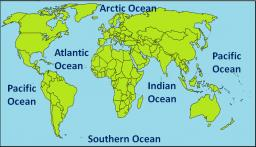Did you find an error or inaccuracy? Feel free to write us. Thank you!Tips to related online calculators
Need help to calculate sum, simplify or multiply fractions? Try our fraction calculator.

## Related math problems and questions:

• Fractions and mixed numerals(a) Convert the following mixed numbers to improper fractions. i. 3 5/8 ii. 7 7/6 (b) Convert the following improper fraction to a mixed number. i. 13/4 ii. 78/5 (c) Simplify these fractions to their lowest terms. i. 36/42 ii. 27/45 2. evaluate the follow
• The EarthThe Earth's surface is 510,000,000 km2. Calculates the radius, equator length, and volume of the Earth, assuming the Earth has the shape of a sphere.
• Decimal expansion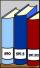Calculate: 2 . 1 + 0 . 10 + 7 . 10000 + 4 . 1000 + 6 . 100 + 0 . 100000 =
• Earth's surface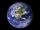The greater part of the earth's surface (r = 6371 km) is covered by oceans; their area is approximately 71% of the Earth's surface. What is the approximate area of the land?
• FractionsSort fractions z1 = (6)/(11); z2 = (10)/(21); z3 = (19)/(22) by its size. Result write as three serial numbers 1,2,3.
• A rectangle 6A rectangle measures 2 4/5 inches by 2 1/5 inches. What is its area?
• The temperatureThe temperature at 1:00 was 10 F. Between 1:00 and 2:00, the temperature dropped 15F. Between 2:00 and 3:00, the temperature rose 3F. What is the temperature at 3:00?
• The perimeter 2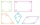The perimeter of the quadrilateral a = 1m b = 14/5m c = 2 3/10m d = 1 4/5m?
• Two walls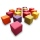Calculate the surface area of a cube in m2 if you know that the area of its two walls is 72 dm2.
• Fraction of a NumberIf 1/2 of 1/3 of 1/4 of 1/5 of a number is 2.5. what is the number?
• Divide 11Divide the product of 4 and 5/8 by 1 1/2. Write your answer as a mixed number.
• Chocolate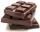How many times do we have to break a chocolate bar composed of 10 × 12 pieces to get the 120 parts?
• Product increased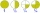When the product of 2/3 and 6/10 is increased by 2/5, the result is?
• Four prismsQuestion No. 1: The prism has the dimensions a = 2.5 cm, b = 100 mm, c = 12 cm. What is its volume? a) 3000 cm2 b) 300 cm2 c) 3000 cm3 d) 300 cm3 Question No.2: The prism base is a rhombus with a side length of 30 cm and a height of 27 cm. The height of t
• Rope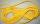Peter has a long rope 2 4/5 meter. Martin has a rope 20 cm longer. What distance ropes achieved if the boys link ropes?
• A bottle 2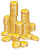A bottle contain 255 coins 1/3 of the coins are £1 cons 110 of the coins are 50p coins the rest of the coins are 20p coins. What is the total value of the coins contained in the bottle.
• Bus driver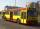The bus driver rides 174 km in the morning, afternoon rides 26 km more. How many kilometers traveled in 5 days if he rides on the same line?